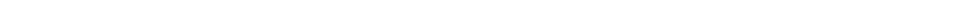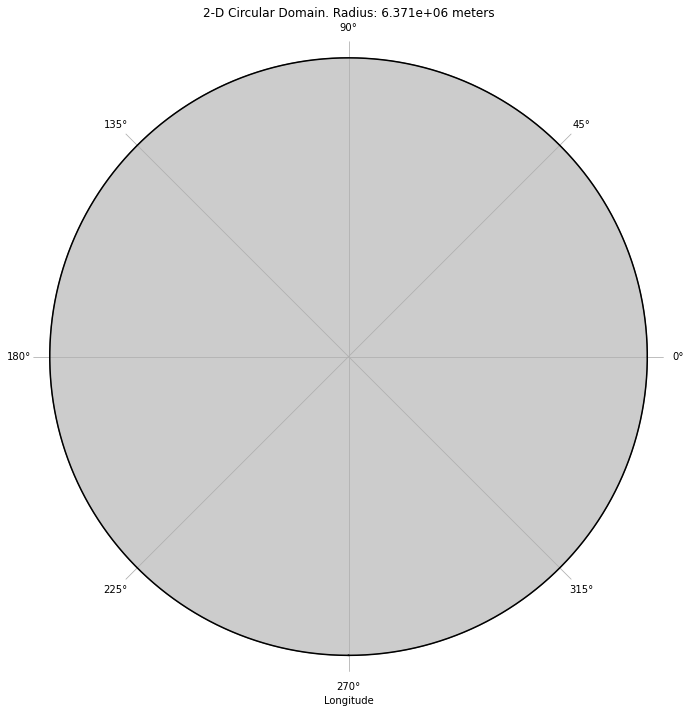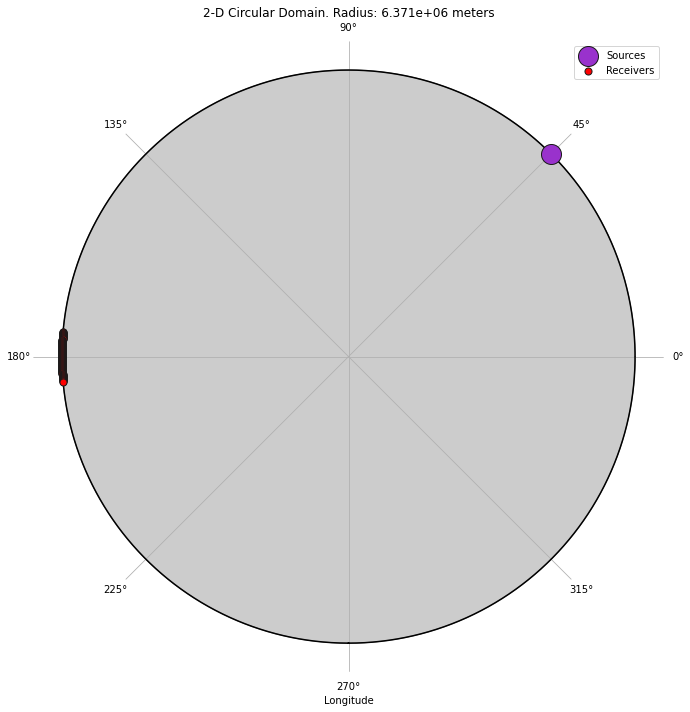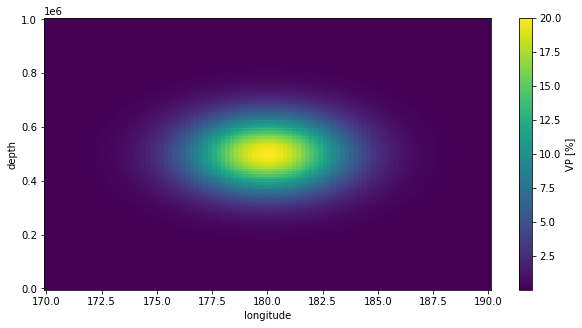Version:

This documentation is not for the latest stable Salvus version.This tutorial is presented as Python code running inside a Jupyter Notebook, the recommended way to use Salvus. To run it yourself you can copy/type each individual cell or directly download the full notebook, including all required files.# 2-D Global-scale simulations w/ heterogeneous models

In this tutorial we'll set up two 2-D teleseismic simulations, with and without a scatter underneath a receiver array. We'll then look at the data we've recorded, and see what types of differences the scatter introduces. First we'll need to import our supporting Python packages.
Copy
import os
import typing

import numpy as np
import xarray as xr

import salvus.namespace as sn
from salvus.project.tools.processing.seismology import (
get_bandpass_filter_processing_function,
)
Now, let's set up our simulation state. Feel free to fiddle with these parameters, with the understanding that simulation costs in 2-D scale with frequency to the power of 3, and memory requirements scale to the power of 2.
n_ranks = 2
resolved_period = 100.0
salvus_flow_site_name = os.environ.get("SITE_NAME", "local")

## Setting up a 2-D global domain in SalvusProject

To keep the problem tractable on small to medium-sized machines, the simulations will be run in 2-D. The first thing to do is to set up our simulation "domain". From this domain, we can create a "project" which will store and organize our real and synthetic data for us.
d = sn.domain.dim2.CircularDomain(radius_in_meter=6371e3)

# !rm -rf proj # Uncomment to restart from scratch.
p = sn.Project.from_domain(path="proj", domain=d, load_if_exists=True)To get a feel for what the domain looks like, we can plot it below. Here we see that the domain is a circle with the radius of Earth. Salvus considers the 2-D circular domain to be a slice through the equator of the equivalent 3-D sphere. This means that latitude values are all $0$, and the azimuthal coordinate is equivalent to longitude.
d.plot()The next step is to add a source and some receivers into the mix. First, let's create a 2-D moment-tensor source that's 10 km below the surface, at a longitude value of 45 degrees. We choose a SideSet... variant of a source here. Accurately locating sources and receivers with respect to deformed mesh surfaces is a nonlinear problem in general. The SideSet... specification here instructs Salvus to solve an optimization problem before any simulations begin, to accurately locate points given a reference surface. Here the "r1" specification implies that the surface of Earth is our coordinate reference.
src = [
sn.simple_config.source.seismology.SideSetMomentTensorPoint2D(
mrr=1e21,
mpp=1e21,
mrp=-1e21,
longitude=45.0,
depth_in_m=10e3,
side_set_name="r1",
)
]
Now let's add some receivers at a teleseismic distance. Here we place an array of 101 receivers which are equidistantly spaced in longitude. The same discussion from above regarding the SideSet... prefix applies here, and a depth of 0 means that the receivers will be placed exactly at the surface.
lons = np.linspace(175.0, 185.0, 101)

recs = [
longitude=l,
depth_in_m=0.0,
side_set_name="r1",
fields=["velocity"],
station_code=f"{_i:06d}",
)
for _i, l in enumerate(lons)
]
Finally, we can add this collection of sources and receivers to our project as an EventCollection. Adding this to our project means that the data will be saved to disk, so we don't need to worry about accidentally overwriting objects we've previously created. Additionally, it allows to shut down the notebook, and come back later to pick up our work.
p.add_to_project(sn.EventCollection.from_sources(sources=src, receivers=recs))
To make sure everything went as expected, let's plot the domain again with the "event" overlaid. If a name is not provided, Salvus will give events consecutive names such as "event_0000", "event_0001", and so on.
p.domain.plot(events=p.events.get("event_0000"))Whether working in Cartesian or spherical coordinates, working with models parameterized on a regular grid provides many benefits. The external xarray package eases this process even further. xarray natively supports reading models saved as CF-compliant NetCDF files, including many of those stored in the IRIS Earth Model Catalogue. Here we'll create a model from scratch, which parameterizes a Gaussian heterogeneity in units of percent perturbation. First, let's initialize the xarray data structure. The model here extends 2000 km from the surface, and spans longitudes 170 to 190. We'll perturb "VP" in this case, and initialize the perturbation array to $0$.
lons = np.linspace(170, 190, 101)
deps = np.linspace(0, 1000e3, 101)
ll, dd = np.meshgrid(lons, deps, indexing="ij")

raw_model = xr.Dataset(
coords={"longitude": lons, "depth": deps},
data_vars={
"VP": (["longitude", "depth"], np.zeros_like(ll), {"units": "%"})
},
attrs={
"geospatial_lon_units": "degrees_east",
"geospatial_vertical_units": "m",
},
)
Now, let's write a little function which will help us add a 2-D Gaussian to our model.
def gaussian_2d(
m: xr.DataArray,
a: float,
c: typing.Tuple[float, float],
s: typing.Tuple[float, float],
) -> xr.DataArray:
"""
Return a 2-D gaussian.

:param m: The xarray model object. Requires longitude and
depth as coordinates.
:param a: Amplitude of the Gaussian.
:param c: Center of the gaussian (c_lon, c_rad).
:param s: Sigma of the gaussian (lon, dep).
"""

dep = m.depth
lon = m.longitude
return a * np.exp(
-(
np.abs(lon - c) ** 2 / (2 * s ** 2)
+ (np.abs(dep - c) ** 2 / (2 * s ** 2))
)
)
Next, let's add the Gaussian to our relative VP model (initialized to zero above). We can then plot the result to get an idea of what the perturbation looks like.
raw_model["VP"] += gaussian_2d(
raw_model.VP, 20, (ll.mean(), dd.mean()), (2.5, 100e3)
)
raw_model.VP.T.plot(figsize=(10, 5))
<matplotlib.collections.QuadMesh at 0x7f7a4232ba90>Finally, with our model defined, let's add it to our project as a "MantleModel". This will transform the regularly-gridded xarray dataset into Salvus' internal representation, ready to be used in a simulation.
While all of this section is specialized for 2-D cylindrical models, the same process applies for 3-D spherical as well as 2- and 3-D Cartesian models.
sm = sn.model.volume.seismology.MantleModel(name="2d_model", data=raw_model)
p.add_to_project(sm)

## Setting up a simulation

Now we're almost ready to fire off our first simulations. Below we define two SimulationConfiurations: one through a 1-D PREM model, and one with our Gaussian blob interpolated as a relative perturbation. The primary difference between each SimulationConfiguration below is the model we'll use. In the first, we pass None for our volume model. In the second, we pass the model we defined above, or "2d_model". The short loop below then defines two distinct simulations, one with and one without the perturbation, and adds them to our project with the names "1d" and "2d" respectively. For a detailed overview of all the settings below, check out this video tutorial.
for name, model in zip(["1d", "2d"], [None, "2d_model"]):

sn.SimulationConfiguration(
name=name,
tensor_order=2,
max_depth_in_meters=6371e3,
elements_per_wavelength=1.0,
min_period_in_seconds=resolved_period,
model_configuration=sn.ModelConfiguration(
background_model=sn.model.background.one_dimensional.BuiltIn(
name="prem_iso_one_crust"
),
volume_models=model,
),
event_configuration=sn.EventConfiguration(
wavelet=sn.simple_config.stf.GaussianRate(
half_duration_in_seconds=2 * resolved_period
),
waveform_simulation_configuration=sn.WaveformSimulationConfiguration(
end_time_in_seconds=2000.0
),
),
)
)
We can now visualize the simulation mesh here in the notebook, which helps to ensure that everything was constructed as expected. Below we visualize the "2d" configuration -- look out for the source and receiver positions, as well as the perturbation we defined in our model. And caution -- the in-notebook mesh visualization is appropriate for small to medium size meshes. If you are working with high frequencies, we recommend you visualize the mesh in Paraview instead.
if resolved_period > 10:
p.viz.nb.simulation_setup("2d")._repr_html_()
[2022-04-30 20:11:18,053] INFO: Creating mesh. Hang on.
Interpolating model: 2d_model